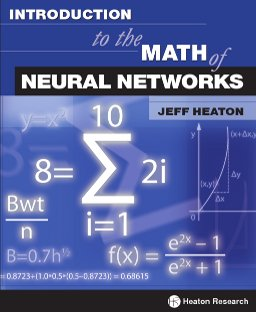[Back to books]

# Introduction to the Math of Neural NetworksTitle:
Introduction to the Math of Neural Networks
ISBN:
9781604390339
Author:
Jeff Heaton
Pages:
112
Status:
Available
Errata:
[Click to View]

Note: Our PDF books contain no DRM and can be printed, copied to multiple computers owned by you, and once downloaded do not require an internet connection.

# Description

This book introduces the reader to the basic math used for neural network calculation. This book assumes the reader has only knowledge of college algebra and computer programming. This book begins by showing how to calculate output of a neural network and moves on to more advanced training methods such as backpropagation, resilient propagation and Levenberg Marquardt optimization. The mathematics needed by these techniques is also introduced.

Mathematical topics covered by this book include first, second, Hessian matrices, gradient descent and partial derivatives. All mathematical notation introduced is explained. Neural networks covered include the feedforward neural network and the self organizing map. This book provides an ideal supplement to our other neural books. This book is ideal for the reader, without a formal mathematical background, that seeks a more mathematical description of neural networks.

# Contents

• Chapter 1: Neural Network Activation: Shows how the output from a neural network is calculated. Before you can see how to train and evaluate a neural network you must understand how a neural network produces its output.

• Chapter 2: Error Calculation: Demonstrates how to evaluate the output from a neural network. Neural networks begin with random weights. Training adjusts these weights to produce meaningful output.

• Chapter 3: Understanding Derivatives: Focuses entirely on a very important Calculus topic. Derivatives, and partial derivatives, are used by several neural network training methods. This chapter will introduce you to those aspects of derivatives that are needed for this book.

• Chapter 4: Training with Backpropagation: Shows you how to apply knowledge from Chapter three towards training a neural network. Backpropagation is one of the oldest training techniques for neural networks. There newer, and much superior, training methods available. However, understanding backpropagation provides a very important foundation for RPROP, QPROP and LMA.

• Chapter 5: Faster Training with RPROP: Introduces resilient propagation (RPROP) which builds upon backpropagation to provide much quicker training times.

• Chapter 6: Weight Initialization: Shows how neural networks are given their initial random weights. Some sets of random weights perform better than others. This chapter looks at several, less than random, weight initialization methods.

• Chapter 7: LMA Training: Introduces the Levenberg Marquardt Algorithm (LMA). LMA is the most mathematically intense training method in this book. LMA sometimes offers very rapid training for a neural network.

• Chapter 8: Self Organizing Maps: Shows how to create a clustering neural network. The SOM can be used to group data. The structure of the SOM is similar to the feedforward neural networks seen in this book.

• Chapter 9: Normalization: Shows how numbers are normalized for neural networks. Neural networks typically require that input and output numbers be in the range of 0 to 1, or -1 to 1. This chapter shows how to transform numbers into that range.